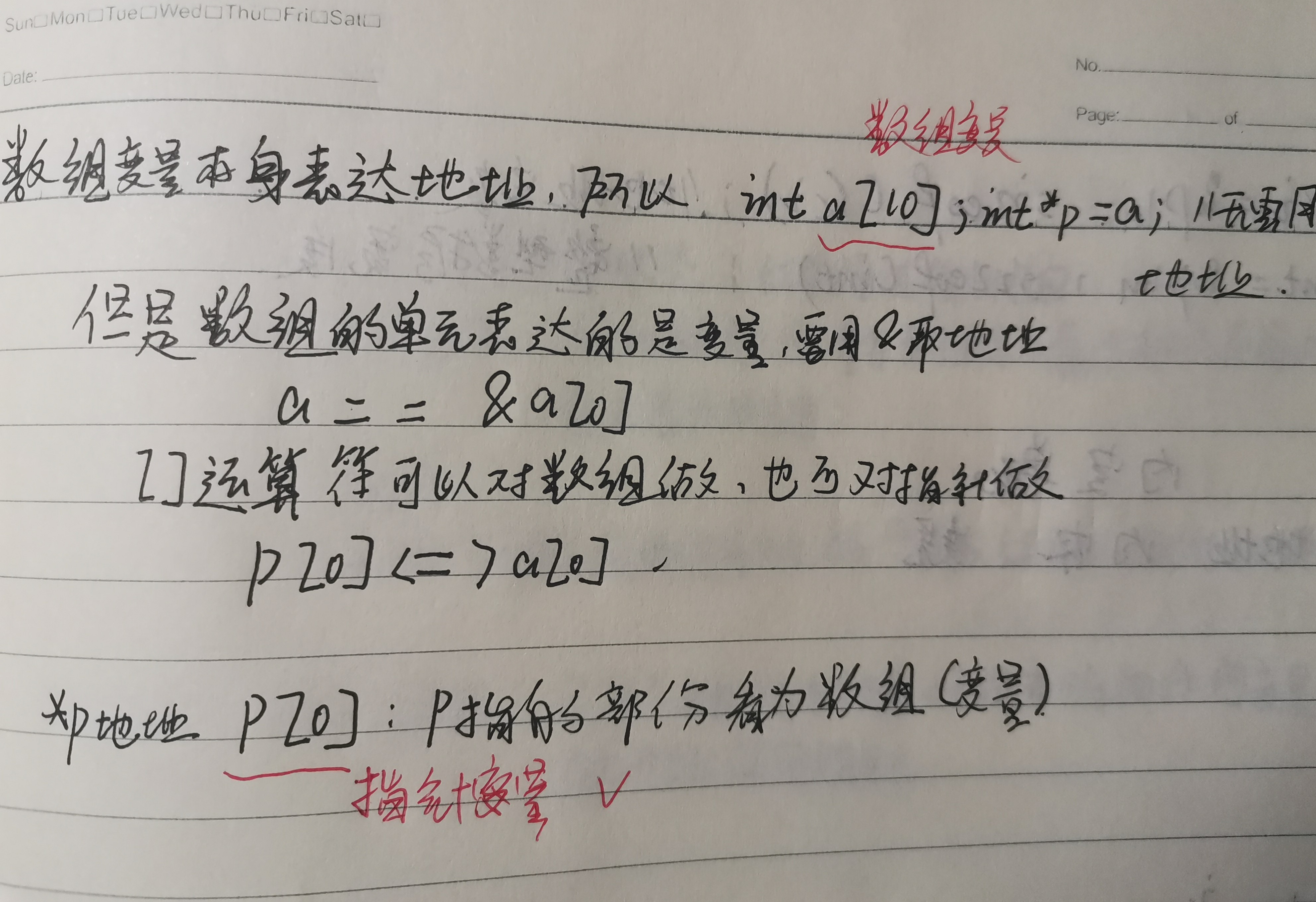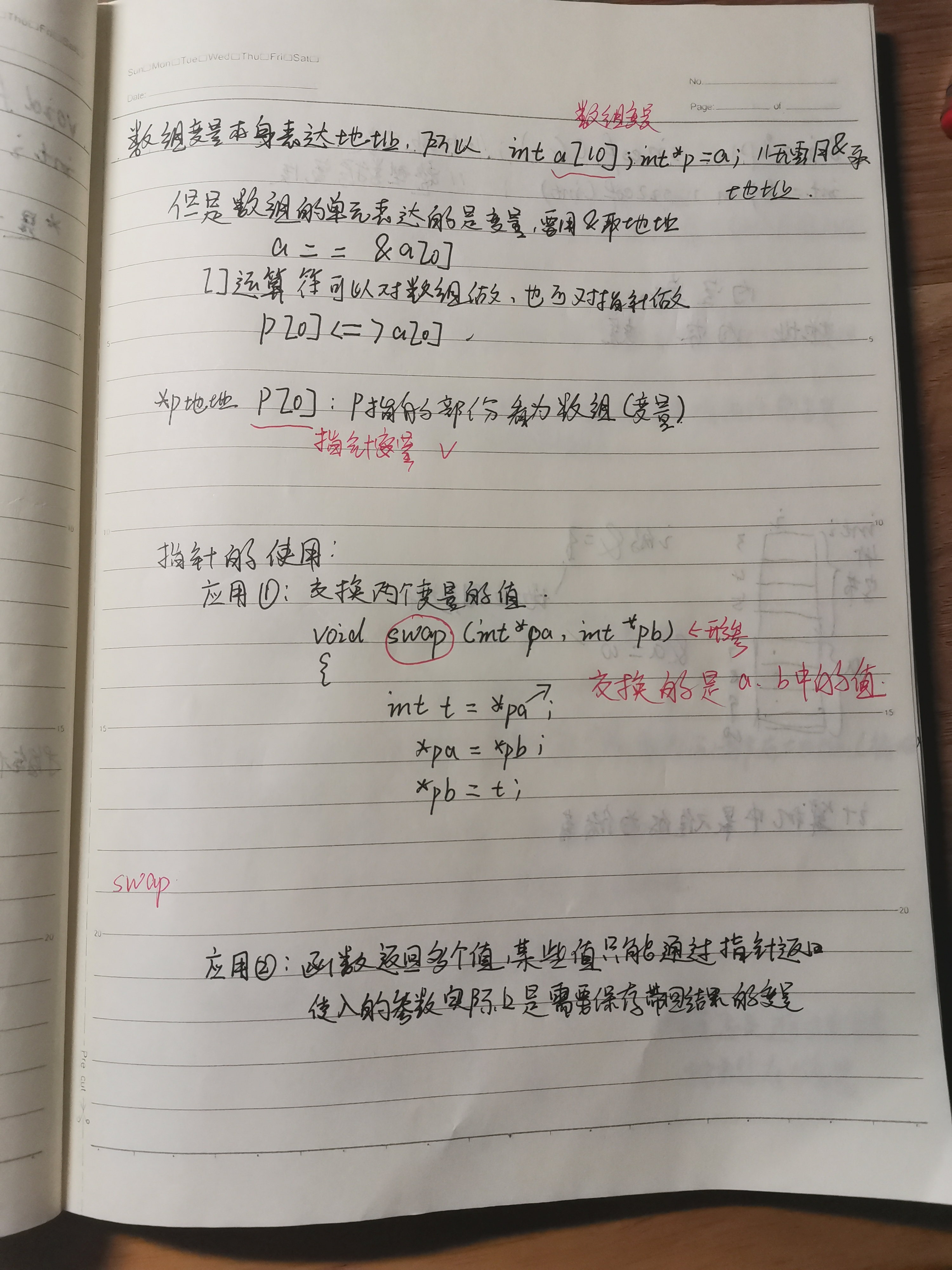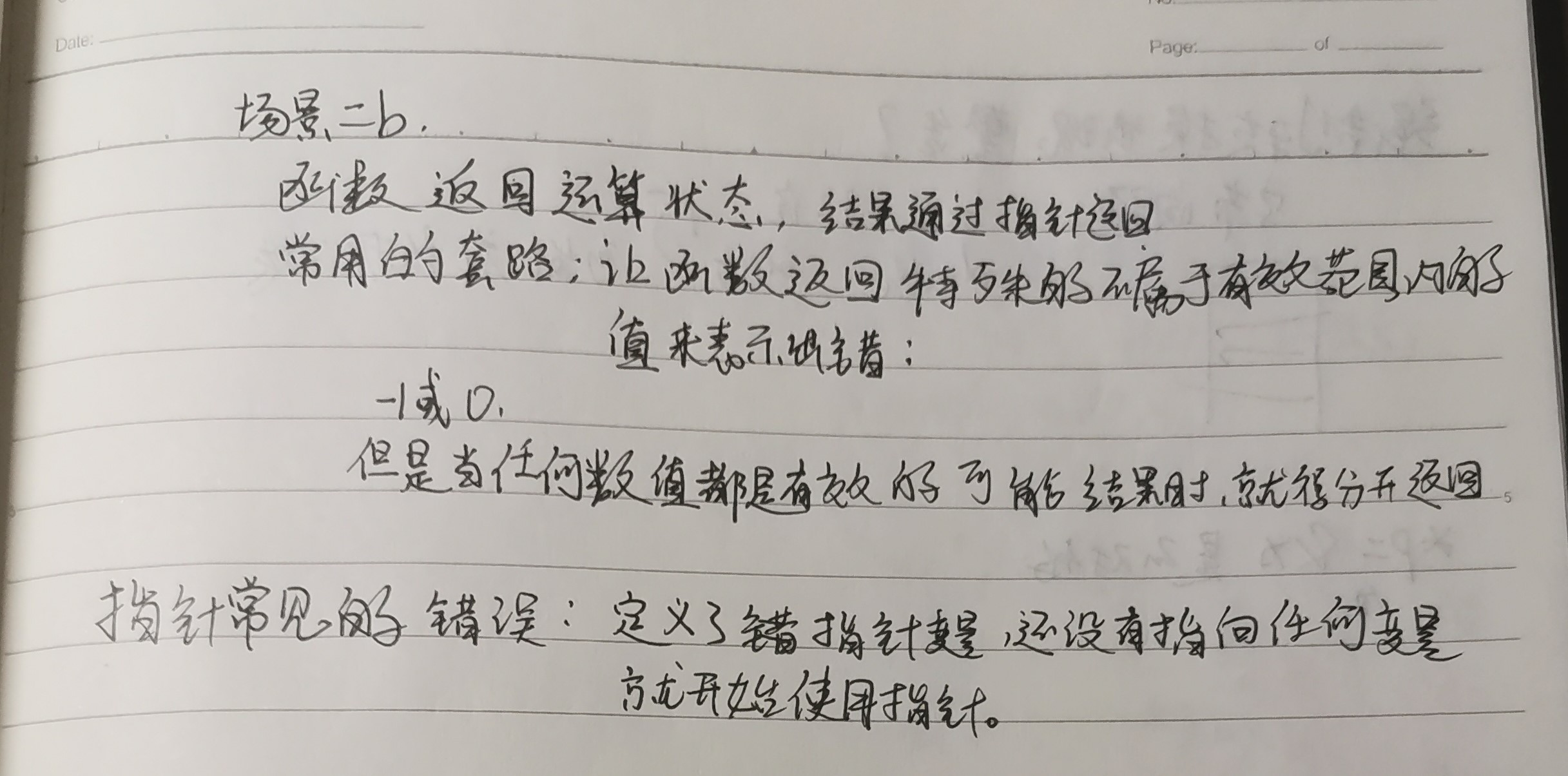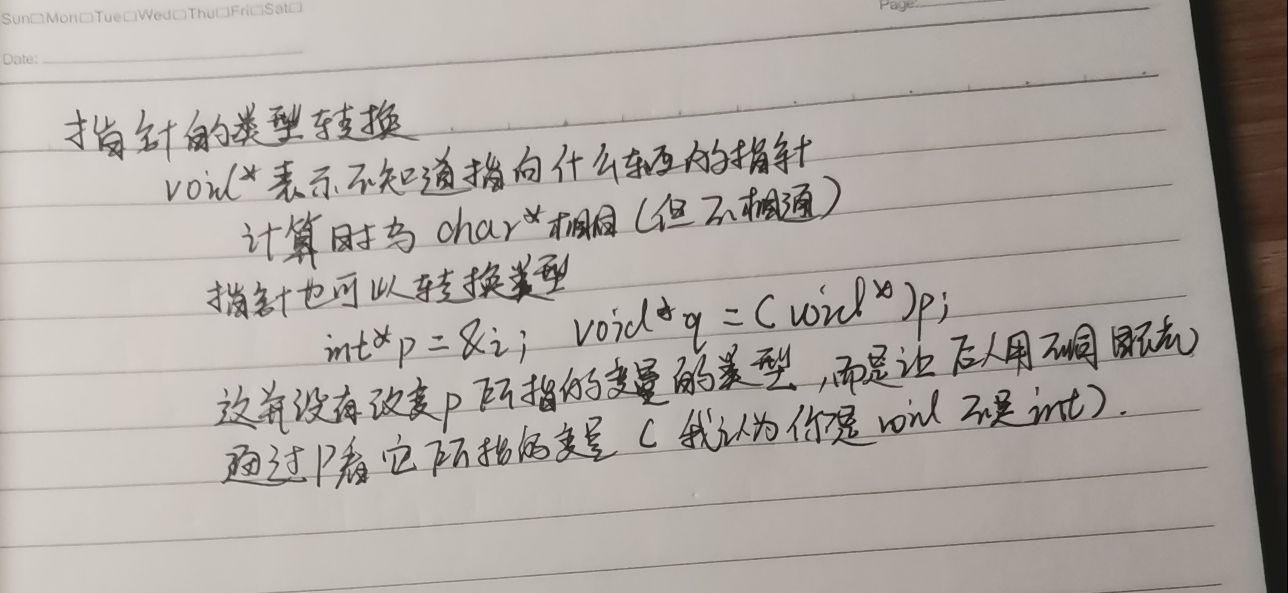# 第二次作业二，代码列表1，null是什么意思，有何用？

2  [Error] 'printf' was not declared in this scope的意思？

“was not declared in this scope”是一个错误信息，在编译的时候会遇到。其含义为标识符在其出现的地方是未被定义的。

3，find的作用？

pta问题

4，对于定义int a,*p=a; 语句p=a+1;和a=a+1;都是合法的吗？

5，下面程序段的运行结果是 6 6 7

  int i,j,k=5, *p;
p= &k;
i= ++(*p);
j=(*p)++;
printf("%d %d %d",i,j,k) ;

6，

void swap(int *a, int *b)

{

int *t;

t=a; a=b; b=t; }

int main() {
int i=2,j=5,*p=&i,*q=&j;

swap(p,q);

printf("%d %d\n",*p,*q);

答；swap函数是用于数值交换的，其中p，q是实参，p是i的地址，q是j的·地址，那*a *b是与p q一至，swap交换后输出 5 2

7

#include <stdio.h>
void find(int *, int, int *, int *);
int main(void)
{
int max, min, a[]={5,3,7,9,2,0,4,1,6,8};
find(a,sizeof(a),&max,&min);
printf("%d,%d\n", max, min);
return 0;
}
void find(int *a, int n, int *max, int *min)
{
int i;
*max=*min=0;　　　　　＜－－－表示的是i吗？
for (i = 1; i < n; i++)
{
if (a[i] > a [*max]) *max = i;
if (a[i] < a [*min]) &min = i;
}

n为数组a的大小，表示数组的大小用sizeof（a）。注意：形参与实参一定要一一对应。

posted @ 2020-03-07 18:08  计科19-2关世雄  阅读(528)  评论(0编辑  收藏  举报